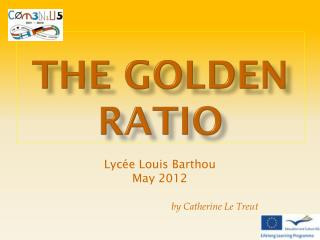DownloadDownload PresentationTHE GOLDEN RATIO

# THE GOLDEN RATIO

Télécharger la présentation## THE GOLDEN RATIO

- - - - - - - - - - - - - - - - - - - - - - - - - - - E N D - - - - - - - - - - - - - - - - - - - - - - - - - - -
##### Presentation Transcript

1. THE GOLDEN RATIO Lycée Louis Barthou May 2012 by Catherine Le Treut

2. A gEomEtriC CUT EUCLID (Greek, -300 ) explains a construction for cutting a line « in extreme and mean ratio"

3. x x + y y CALCULATE THE GOLDEN RATIO x length of the medium segment y length of the small segment The name of Greek sculptor Phidias phi is used to symbolize the golden ratio

4. THE GOLDEN RATIO IN HISTORY In 1509, FRA LUCA PACIOLI, Italian mathematician and Franciscan friar talk about it in his book: « De divinaproportione » Leonardo da Vinci illustrate the book and name the ratio: « sectiaaurea » In 1596, Johannes KEPLER (German astronomer) talk about it again as a “jewel of geometry”. During 19th century, the philosopher Adolf ZEIZING name this ratio the “golden section” and look for finding it in Roman and Greek temples (the Parthenon, ……) , Greek sculptures, European cathedrals, ….. In 1932, Prince MatilaGhyka, Romanian diplomat et mathematician, call it the golden ratio and try to find it everywhere ……

5. THE GOLDEN RECTANGLE It can be shown with the Pythagorean theorem : ABCD is a square, I middle of [AB] , E a point of the half-line [IB) verifying IC=IE. Rectangles AEFD and BEFC are golden rectangles, the ratio of their sides is equal to

6. The gOLden spiral So, we can build series of golden rectangles and therefore plot a golden spiral. That spiral can be found in some shells.

7. The golden triangles They are isosceles triangles, such as the ratio between two sides is equal to . There are two types:

8. THE REGULAR PENTAGON Each angle is equal to 108°, it is made up of golden triangles and, if the sides length is equal to 1, its diagonal is equal to

9. THE PENTAGRAM The addition of a regular pentagon and a star pentagon gives the pentagram. In the center of this pentagram, we can build and other pentagram and so on…...This figure was the emblem of the Pythagoreans.

10. A continued fraction The most irrational amongst irrational numbers ….

11. The golden ratio in art Many people looked for the golden ratio into art works Is the Parthenon design really based upon the golden ratio ? Drawings recommended by those who sustain that theory seem complicated …

12. The golden ratio in art Seurat’s painting Based upon the golden ratio? Or by dividing in two, then in two, the in two …. i.e. in

13. Someartits have used the golden ratio in plentyoftheir art works The golden ratio in art Jacques Villon, french cubistpainter The famousarchitect Le Corbusier whenhecreates the «  cité Radieuse » in Marseille

14. The golden ratio in art What about in the comic books ? The golden points in a rectangle are defined by dividing each side according to the golden ratio. Please check that these plots define a golden point Could You find a golden point here? « The crab with the golden claws ». Hergé

15. FIBONNACCI sequence • Leonardo Fibonnacci (12e/13e century) • Italian Mathematician, also named Leonardo Pisano • He has worked on the following sequence of natural numbers • The first two equal 1 • A term can be obtained with adding the two immediately before 1 1 2 3 5 8 13 21 34 55 89 144 …. The sequence of the ratios of two following terms tends towards the golden ratio

16. Fibonnacci has used a population of rabbits as an example for his sequence. • For each step: • A couple of baby rabbits becomes a couple of adult rabbits • A couple of adults doesn’t die, and gives birth to a couple of babies FIBONNACCI’s rabbits

17. The golden ratio in nature • The golden angle divides a circle into two arcs whose ratio is . As the most « irrational number », it’s the one which allows the optimal filling of space for the formation of plants, pine cones, the position of leaves around a stem…. • If you count the number of spirals in a sunflower or a pine cone, in one direction and then in the other, you will find two following terms of the Fibonnacci’s sequence. Geospacemodelling

18. Golden ratio at the cathedrals time Cathedrals or abbeys builders used length measures inspired by the proportions of the human body. These measures could be found on the builders’ stick, named « la QUINE ». One « ligne » is 0,2247 cm (the diameter of a barley grain…) Paume 34 lignes 7,64cm Palme 55 lignes 12,36 cm Empan 89 lignes 20,00 cm Pied 144 lignes 32,36 cm Coudée 233 lignes 52,36 cm You can find again the terms of the Fibonnacci’s sequence

19. T N G A golden man? Some people say that the ideal proportions for a man (or a woman) follow a golden ratio : So try! ST : height SN : distance ground –belly button NT : distance belly button-top of the head CONCLUSION ?

20. Heimildir • PWR: THE GOLDEN RATIO http://www.europeansharedtreasure.eu/detail.php?id_project_base=2011-1-FR1-COM06-24441